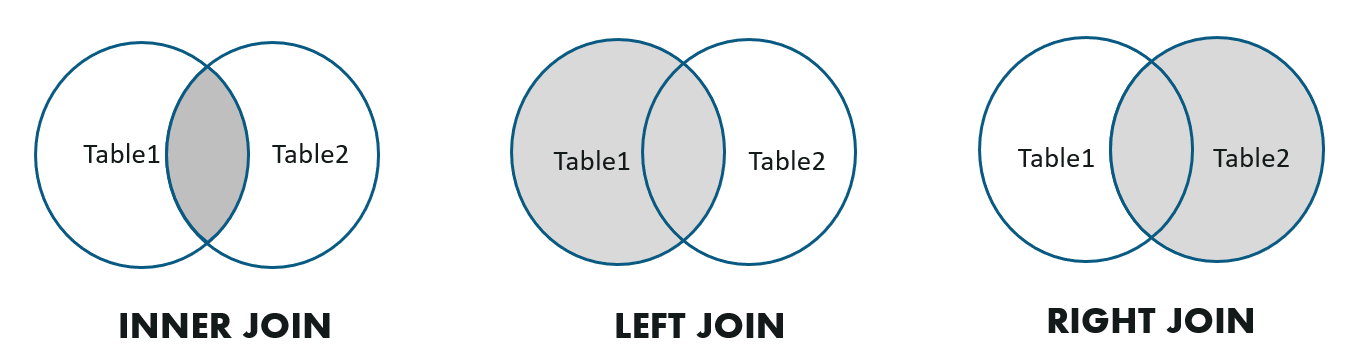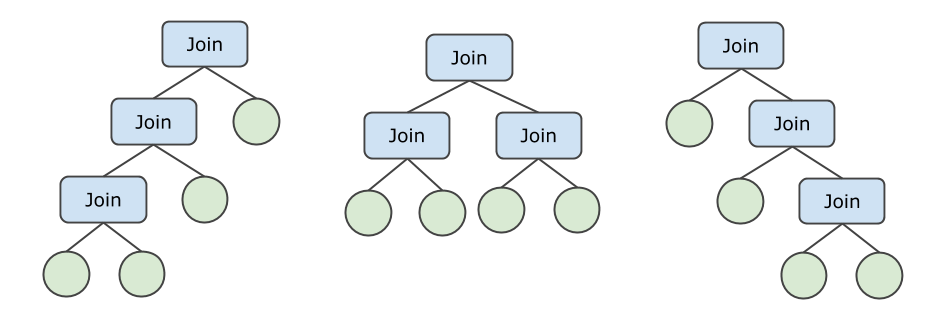ET大脑

API与工具

# Join 与子查询的优化和执行

## 基本概念

Join 是 SQL 查询中常见的操作，逻辑上说，它的语义等价于将两张表做笛卡尔积，然后根据用户提供的过滤条件过过滤，留下满足条件的数据行。Join 多数情况下是依赖等值条件做的 Join，即 Equi-Join，用来根据某个特定列的值连接两张表的数据。

## Join 类型

DRDS 支持以下 3 种常见的 Join 类型：Inner Join，Left Outer Join，Right Outer Join``/* Inner Join */``SELECT * FROM A, B WHERE A.key = B.key;``/* Left Outer Join */``SELECT * FROM A LEFT JOIN B ON A.key = B.key;``/* Right Outer Join */``SELECT * FROM A RIGHT OUTER JOIN B ON A.key = B.key;``

``/* Semi Join - 1 */``SELECT * FROM Emp WHERE Emp.DeptName IN ( ``   SELECT DeptName FROM Dept``)`` /* Semi Join - 2 */``SELECT * FROM Emp WHERE EXISTS (``  SELECT * FROM Dept WHERE Emp.DeptName = Dept.DeptName``)``/* Anti Join - 1 */``SELECT * FROM Emp WHERE Emp.DeptName NOT IN ( ``   SELECT DeptName FROM Dept``)`` /* Anti Join - 2 */``SELECT * FROM Emp WHERE NOT EXISTS (``  SELECT * FROM Dept WHERE Emp.DeptName = Dept.DeptName``)``

## Join 算法

### Nested-Loop Join (NLJoin)

Nested-Loop Join 通常用于非等值的 Join。它的工作方式如下：

1. 拉取内表（右表，通常是数据量较小的一边）的全部数据，缓存到内存中
2. 遍历外表数据，对于外表的每行：
• 对于每一条缓存在内存中的内表数据
• 构造结果行，并检查是否满足 Join 条件，如果满足条件则输出

``> EXPLAIN SELECT * FROM partsupp, supplier WHERE ps_suppkey < s_suppkey;``NlJoin(condition="ps_suppkey < s_suppkey", type="inner")``  Gather(concurrent=true)``    LogicalView(tables="partsupp_[0-7]", shardCount=8, sql="SELECT * FROM `partsupp` AS `partsupp`")``  Gather(concurrent=true)``    LogicalView(tables="supplier_[0-7]", shardCount=8, sql="SELECT * FROM `supplier` AS `supplier`")``

``/*+TDDL:NL_JOIN(outer_table, inner_table)*/ SELECT ...``

``/*+TDDL:NL_JOIN((outer_table_a, outer_table_b), (inner_table_c, inner_table_d))*/ SELECT ...``

### Hash Join

Hash Join 是等值 Join 最常用的算法之一。它的原理如下，

1. 拉取内表（右表，通常是数据量较小的一边）的全部数据，写进内存中的哈希表
2. 遍历外表数据，对于外表的每行：
• 根据等值条件 Join Key 查询哈希表，取出 0-N 匹配的行（Join Key 相同）
• 构造结果行，并检查是否满足 Join 条件，如果满足条件则输出

``> EXPLAIN SELECT * FROM partsupp, supplier WHERE ps_suppkey = s_suppkey;``HashJoin(condition="ps_suppkey = s_suppkey", type="inner")``  Gather(concurrent=true)``    LogicalView(tables="partsupp_[0-7]", shardCount=8, sql="SELECT * FROM `partsupp` AS `partsupp`")``  Gather(concurrent=true)``    LogicalView(tables="supplier_[0-7]", shardCount=8, sql="SELECT * FROM `supplier` AS `supplier`")``

Hash Join 常出现在 Join 数据量较大的复杂查询、且无法通过索引 Lookup 来改善，这种情况下，Hash Join 是最优的选择。例如上面的例子中，partsupp 表和 supplier 表均为全表扫描，数据量较大，适合使用 HashJoin。

``/*+TDDL:HASH_JOIN(table_outer, table_inner)*/ SELECT ...``

### Lookup Join (BKAJoin)

Lookup Join 是另一种常用的等值 Join 算法，常用于数据量较小的情况。它的原理如下，

1. 遍历外表（左表，通常是数据量较小的一边）数据，对于外表中的每批（例如 1000 行）数据
1. 将这一批数据的 Join Key 拼成一个 `IN (....)` 条件，加到内表的查询中
2. 执行内表查询，得到 Join 匹配的行
3. 借助哈希表，为外表的每行找到匹配的内表行，组合并输出

``> EXPLAIN SELECT * FROM partsupp, supplier WHERE ps_suppkey = s_suppkey AND ps_partkey = 123;``BKAJoin(condition="ps_suppkey = s_suppkey", type="inner")``  LogicalView(tables="partsupp_3", sql="SELECT * FROM `partsupp` AS `partsupp` WHERE (`ps_partkey` = ?)")``  Gather(concurrent=true)``    LogicalView(tables="supplier_[0-7]", shardCount=8, sql="SELECT * FROM `supplier` AS `supplier` WHERE (`s_suppkey` IN ('?'))")``

Lookup Join 通常用于外表数据量较小的情况，例如上面的例子中，左表 partsupp 由于存在 `ps_partkey = 123` 的过滤条件，仅有几行数据。此外，右表的 `s_suppkey IN ( ... )` 查询命中了主键索引，这也使得 Lookup Join 的查询代价进一步降低。

``/*+TDDL:BKA_JOIN(table_outer, table_inner)*/ SELECT ...``

### Sort-Merge Join

Sort-Merge Join 是另一种等值 Join 算法，它依赖左右两边输入的顺序，必须按 Join Key 排好序。

1. 开始 Sort-Merge Join 之前，输入端必须排好序（借助 MergeSort 或 MemSort）
2. 比较当前左右表输入的行，并按以下方式操作，不断消费左右两边的输入：
• 如果左表的 Join Key 较小，则消费左表的下一条数据
• 如果右表的 Join Key 较小，则消费左表的下一条数据
• 如果左右表 Join Key 相等，说明获得了 1 条或多条匹配，检查是否满足 Join 条件并输出

``> EXPLAIN SELECT * FROM partsupp, supplier WHERE ps_suppkey = s_suppkey ORDER BY s_suppkey;``SortMergeJoin(condition="ps_suppkey = s_suppkey", type="inner")``  MergeSort(sort="ps_suppkey ASC")``    LogicalView(tables="QIMU_0000_GROUP,QIMU_0001_GROUP.partsupp_[0-7]", shardCount=8, sql="SELECT * FROM `partsupp` AS `partsupp` ORDER BY `ps_suppkey`")``  MergeSort(sort="s_suppkey ASC")``    LogicalView(tables="QIMU_0000_GROUP,QIMU_0001_GROUP.supplier_[0-7]", shardCount=8, sql="SELECT * FROM `supplier` AS `supplier` ORDER BY `s_suppkey`")``

Sort-Merge Join，由于需要额外的排序步骤，通常 Sort-Merge Join 并不是最优的。但是，某些情况下用户查询恰好也需要按 Join Key 排序（上面的例子），这时候使用 Sort-Merge Join 是较优的选择。

``/*+TDDL:SORT_MERGE_JOIN(table_a, table_b)*/ SELECT ...``

## Join 顺序• 当（未下推的）N 较小时，采取 Bushy 枚举策略，会在所有 Join 顺序中选出最优的计划
• 当（未下推的）表的数量较多时，采取 Zig-Zag（锯齿状） 或 Left-Deep（左深树）的枚举策略，选出最优的 Zig-Zag 或 Left-Deep 执行计划，以减少枚举的次数和代价

DRDS 使用基于代价的优化器（Cost-based Optimizer，CBO）选择出总代价最低的 Join 顺序。详情参见查询优化器介绍

## 子查询

``/* 例子：非关联子查询 */``SELECT * FROM lineitem WHERE l_partkey IN (SELECT p_partkey FROM part);``/* 例子：关联子查询（l_suppkey 是关联项） */``SELECT * FROM lineitem WHERE l_partkey IN (SELECT ps_partkey FROM partsupp WHERE ps_suppkey = l_suppkey);``

DRDS 子查询支持绝大多数的子查询写法，具体参见“SQL 手册”。

``> EXPLAIN SELECT * FROM lineitem WHERE l_partkey IN (SELECT ps_partkey FROM partsupp WHERE ps_suppkey = l_suppkey);``SemiHashJoin(condition="l_partkey = ps_partkey AND l_suppkey = ps_suppkey", type="semi")``  Gather(concurrent=true)``    LogicalView(tables="lineitem_[0-7]", shardCount=8, sql="SELECT * FROM `lineitem` AS `lineitem`")``  Gather(concurrent=true)``    LogicalView(tables="partsupp_[0-7]", shardCount=8, sql="SELECT `ps_partkey`, `ps_suppkey` FROM `partsupp` AS `partsupp`")``
``> EXPLAIN SELECT p_partkey, (``      SELECT COUNT(ps_partkey) FROM partsupp WHERE ps_suppkey = p_partkey``      ) supplier_count FROM part;``Project(p_partkey="p_partkey", supplier_count="CASE(IS NULL(\$10), 0, \$9)", cor=[\$cor0])``  HashJoin(condition="p_partkey = ps_suppkey", type="left")``    Gather(concurrent=true)``      LogicalView(tables="part_[0-7]", shardCount=8, sql="SELECT * FROM `part` AS `part`")``    Project(count(ps_partkey)="count(ps_partkey)", ps_suppkey="ps_suppkey", count(ps_partkey)2="count(ps_partkey)")``      HashAgg(group="ps_suppkey", count(ps_partkey)="SUM(count(ps_partkey))")``        Gather(concurrent=true)``          LogicalView(tables="partsupp_[0-7]", shardCount=8, sql="SELECT `ps_suppkey`, COUNT(`ps_partkey`) AS `count(ps_partkey)` FROM `partsupp` AS `partsupp` GROUP BY `ps_suppkey`")``

``> EXPLAIN SELECT * FROM lineitem WHERE l_partkey IN (SELECT ps_partkey FROM partsupp WHERE ps_suppkey = l_suppkey) OR l_partkey IS NOT``Filter(condition="IS(in,[\$1]) OR l_partkey < ?0")``  Gather(concurrent=true)``    LogicalView(tables="QIMU_0000_GROUP,QIMU_0001_GROUP.lineitem_[0-7]", shardCount=8, sql="SELECT * FROM `lineitem` AS `lineitem`")``>> individual correlate subquery : 29612489``Gather(concurrent=true)``  LogicalView(tables="QIMU_0000_GROUP,QIMU_0001_GROUP.partsupp_[0-7]", shardCount=8, sql="SELECT * FROM (SELECT `ps_partkey` FROM `partsupp` AS `partsupp` WHERE (`ps_suppkey` = `l_suppkey`)) AS `t0` WHERE (((`l_partkey` = `ps_partkey`) OR (`l_partkey` IS NULL)) OR (`ps_partkey` IS NULL))")``